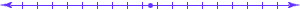### CS 302 Programming Project.

#### Using Dijkstra's Algorithm to Solve the Single Source Shortest Path Problem

• You will solve the single source shortest path problem for a directed weighted graph, with no negative edges, using Dijkstra's Algorithm.
• Read a directed weighted graph G from an input file.
• Here is a small graph.
• The first line of the file contains n, the number of vertices of G. The names of the vertices are the integers from 1 to n.
• Each remaining line of the graph is a list of integers separated by spaces, and represents the list of outneighbors of one vertex.
• The first number in the line is the name of the vertex.
• After that, there is a sequence of pairs, consisting of a vertex name and a weight.
• The source will be vertex 1.
• You are to print a shortest path from the source to each other vertex.
• Possible output if the input is the small graph.
• There will be no more than 1000 vertices in the graph.
• The graph will be sparse.
• Here is a larger graph. If you write Dijkstra's algorithm properly, your computer will solve that graph in "no time."
• If you try to solve that large graph using the Bellman-Ford algorithm, it will take a long time. If you try to solve it using the Floyd-Warshall algorithm, you could starve to death waiting for it to finish.